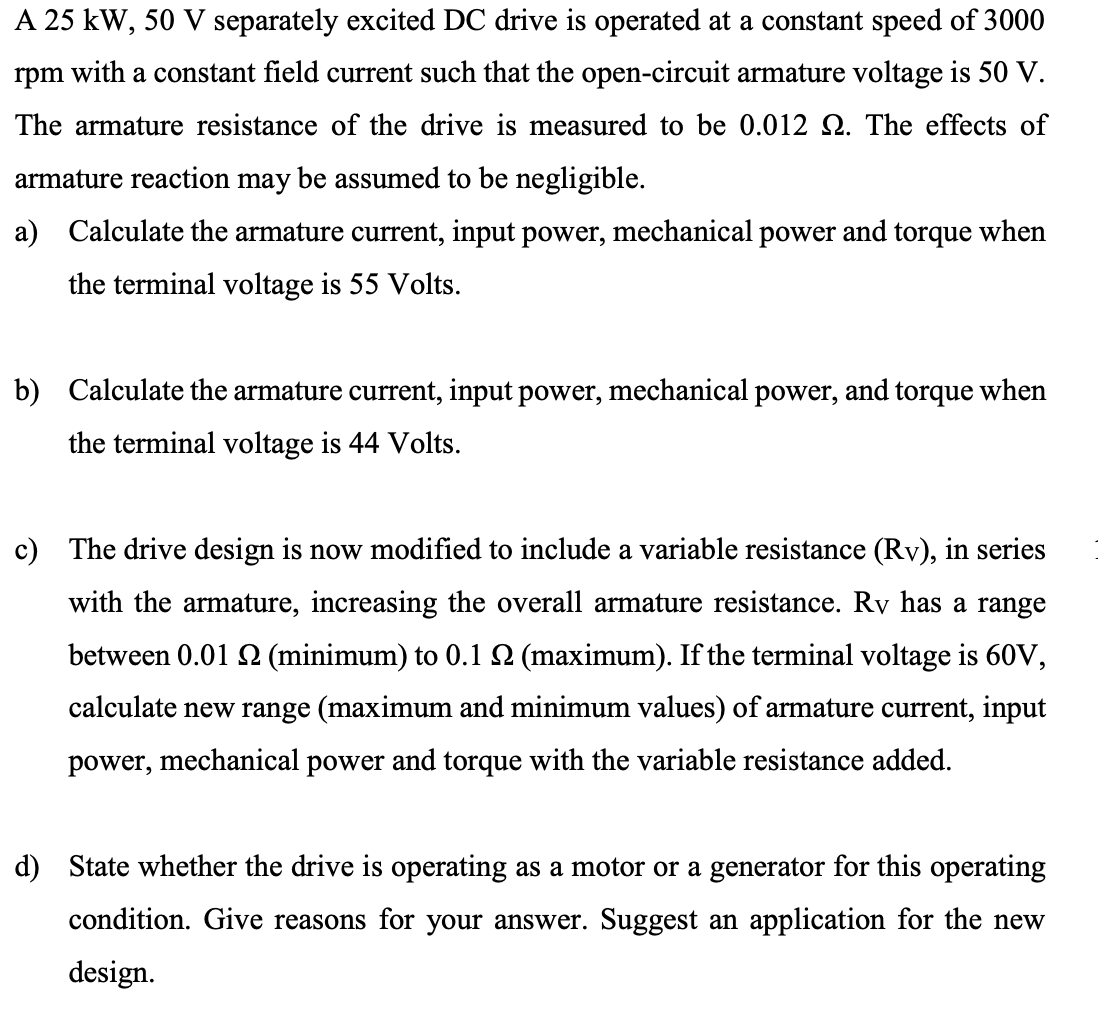# Question Solved1 AnswerA 25 kW, 50 V separately excited DC drive is operated at a constant speed of 3000 rpm with a constant field current such that the open-circuit armature voltage is 50 V. The armature resistance of the drive is measured to be 0.012 12. The effects of armature reaction may be assumed to be negligible. a) Calculate the armature current, input power, mechanical power and torque when the terminal voltage is 55 Volts. b) Calculate the armature current, input power, mechanical power, and torque when the terminal voltage is 44 Volts. c) The drive design is now modified to include a variable resistance (Rv), in series with the armature, increasing the overall armature resistance. Ry has a range between 0.01 12 (minimum) to 0.1 12 (maximum). If the terminal voltage is 60V, calculate new range (maximum and minimum values) of armature current, input power, mechanical power and torque with the variable resistance added. d) State whether the drive is operating as a motor or a generator for this operating condition. Give reasons for your answer. Suggest an application for the new design.C6SZBS The Asker · Electrical EngineeringTranscribed Image Text: A 25 kW, 50 V separately excited DC drive is operated at a constant speed of 3000 rpm with a constant field current such that the open-circuit armature voltage is 50 V. The armature resistance of the drive is measured to be 0.012 12. The effects of armature reaction may be assumed to be negligible. a) Calculate the armature current, input power, mechanical power and torque when the terminal voltage is 55 Volts. b) Calculate the armature current, input power, mechanical power, and torque when the terminal voltage is 44 Volts. c) The drive design is now modified to include a variable resistance (Rv), in series with the armature, increasing the overall armature resistance. Ry has a range between 0.01 12 (minimum) to 0.1 12 (maximum). If the terminal voltage is 60V, calculate new range (maximum and minimum values) of armature current, input power, mechanical power and torque with the variable resistance added. d) State whether the drive is operating as a motor or a generator for this operating condition. Give reasons for your answer. Suggest an application for the new design.
More
Transcribed Image Text: A 25 kW, 50 V separately excited DC drive is operated at a constant speed of 3000 rpm with a constant field current such that the open-circuit armature voltage is 50 V. The armature resistance of the drive is measured to be 0.012 12. The effects of armature reaction may be assumed to be negligible. a) Calculate the armature current, input power, mechanical power and torque when the terminal voltage is 55 Volts. b) Calculate the armature current, input power, mechanical power, and torque when the terminal voltage is 44 Volts. c) The drive design is now modified to include a variable resistance (Rv), in series with the armature, increasing the overall armature resistance. Ry has a range between 0.01 12 (minimum) to 0.1 12 (maximum). If the terminal voltage is 60V, calculate new range (maximum and minimum values) of armature current, input power, mechanical power and torque with the variable resistance added. d) State whether the drive is operating as a motor or a generator for this operating condition. Give reasons for your answer. Suggest an application for the new design.